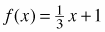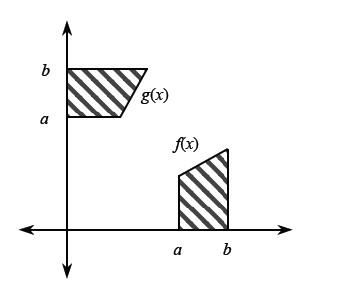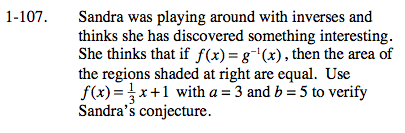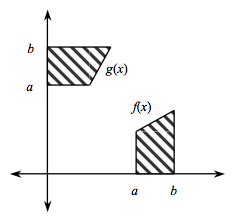### Home > CALC > Chapter 1 > Lesson 1.3.1 > Problem1-107

1-107.
1. Sandra was playing around with inverses and thinks she has discovered something interesting. She thinks that if f(x) = g−1 (x), then the area of the regions shaded below are equal. Usewith a = 3 and b = 5 to verify Sandra's conjecture. Homework Help ✎$\text{If }f(x)=\frac{1}{3}x+1\text{ then }f^{-1}(x)=3(x-1)$

Substitute in the values for a and b. Are the values equal?